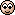A glass tube filled with mercury

ValeForce46

Homework Statement
A glass tube with a section of $A= 10^{-4} m^2$ and length $l=1.14 m$ is closed at one end and open at the other end. Inside the tube there's a mercury's column $l_2=0.3 m$. When the tube is placed horizontally, the columns of air on the right and left of the column of mercury have the same length $l_1=l_3=0.42 m$. The tube is now placed vertically with the open end to the top. Determine the length of the column of air $l_1'$ of the closed end. How long the length $l_1''$ of air would be if the tube was closed before it was placed vertically? Assume temperature constant during every process, air is a perfect gas and $ρ_{Hg}=13.6 ρ_{air}$.
Homework Equations
Boyle's law: $P*V=constant$I guess I have to find out something when the tube is on the horizontal.
What force should I consider? Is the pressure of the air inside still $p_{atm}$?
When it is on the vertical, the equation is something like this?
$P_{Hg}+p_{atm}*A=p_{atm}*A+ρ_{air}*A*(l_1-l_1')*g$ with $P_{Hg}=ρ_{Hg}*A*l_2*g$ weight of the column of mercury.
Or maybe I shouldn't face this problem considering the force...?

Related Introductory Physics Homework Help News on Phys.org

haruspex

Homework Helper
Gold Member
2018 Award
Please try to justify your equation. (I fail to see how the weight of air in the closed section comes into it.)

ValeForce46

That was just an attempt, I don't even think that is right.
I considered as downward forces the weight of the column of mercury $P_{Hg}$ and the atmospheric pressure times the section $p_{atm}*A$.
I considered as upward forces the bouyant force $ρ_{air}∗A∗(l1−l′1)∗g$ and the pressure of the air in the closed section. Is it right to consider the bouyant force? What about the pressure of the closed section?
I fail to see how the weight of air in the closed section comes into it.
Really, I didn't even considered it...haruspex

Homework Helper
Gold Member
2018 Award
Is it right to consider the bouyant force?
no. (This is where you seemed to be doing something with weight of air.)
What about the pressure of the closed section?
Indeed, what about it? What law tells you how the shrinkage in its length leads to greater pressure?

ValeForce46

What law tells you how the shrinkage in its length leads to greater pressure?
Boyle's law. My main dubt is: can I consider the initial pressure of the closed section $p_{atm}$? Why?
In such case, it would be $p_{atm}*A*l_1=p_{f}*A*(l_1-l_1')$
But I still need something else. Where should I look for it? Do I have to adjust the equation of forces (when the tube is on the vertical)?

haruspex

Homework Helper
Gold Member
2018 Award
can I consider the initial pressure of the closed section $p_{atm}$? Why?
Yes, because while horizontal any difference from atmospheric pressure would have caused the mercury to move.
In such case, it would be $p_{atm}*A*l_1=p_{f}*A*(l_1-l_1')$
Doesn’t look right to me.
Always worth checking trivial special cases. What does your equation give if l1 doesn't change?

Last edited:

ValeForce46

Always worth checking trivial special cases. What does your equation give if l1 doesn't change?
It gives $p_{atm}=p_f$. Why is this wrong? If $l_1'$ doesn't change, the air would take the same volume.
Anyways, how should I apply Boyle's law?

EDIT:
well, now I got it: $p_{atm}*A*l_1=p_f*A*l_1'$.
What is the next step?

Last edited:

haruspex

Homework Helper
Gold Member
2018 Award
It gives $p_{atm}=p_f$. Why is this wrong? If $l_1'$ doesn't change, the air would take the same volume.
Anyways, how should I apply Boyle's law?

EDIT:
well, now I got it: $p_{atm}*A*l_1=p_f*A*l_1'$.
What is the next step?
Write the force balance equation for the mercury using pf.

ValeForce46

Is this right?
$ρ_{Hg}*A*l_2*g+p_{atm}*A=p_f*A$
The first term is the weight of mercury. (On the homework statement I did a mistake $ρ_{Hg}=13.6*ρ_{H2O}$)
If yes, then $l_1'=0.3 m$.

haruspex

Homework Helper
Gold Member
2018 Award
Is this right?
$ρ_{Hg}*A*l_2*g+p_{atm}*A=p_f*A$
The first term is the weight of mercury. (On the homework statement I did a mistake $ρ_{Hg}=13.6*ρ_{H2O}$)
If yes, then $l_1'=0.3 m$.
Correct.
The easiest way is to start with atmospheric pressure as 0.76m of Hg. The 0.3m in the tube is going to increase the pressure to 1.06m, i.e. in the ratio 106/76. So the volume of air is reduced in that ratio: (76/106)0.42=0.3.

•ValeForce46

ValeForce46

For the second part (the tube is completely closed before it is on the vertical), do I have to repeat the reasoning I did before?
Now, when the air under the mercury is compressed, the air above the mercury expands.
Boyle's Law:
$p_{atm}*A*l_1=p_1*A*(l_1-x)$ for the air under the mercury
$p_{atm}*A*l_3=p_3*A*(l_3+x)$ for the air above the mercury
and by the force balance equation:
$ρ_{Hg}∗A∗l_2∗g+p_3*A=p_1*A$
3 equation and 3 unknows $p_1$, $p_3$ and $x$. Then I can find $l_1''=l_1-x$
Let me know if this is right please.
Just one last thing, why can I consider the length of mercury constant? I did it for the first part of the problem too.

haruspex

Homework Helper
Gold Member
2018 Award
Let me know if this is right please.
Looks good.
why can I consider the length of mercury constant?
Why would it change (by more than a minuscule amount)?

•ValeForce46

ValeForce46

...Yes, actually there's no reason to change, in this problem. Thanks a lotTSny

Homework Helper
Gold Member
...Yes, actually there's no reason to change, in this problem. Thanks a lotYes, Mercury is highly incompressible. Jupiter however....(sorry!)

•collinsmark and ValeForce46

"A glass tube filled with mercury"

Physics Forums Values

We Value Quality
• Topics based on mainstream science
• Proper English grammar and spelling
We Value Civility
• Positive and compassionate attitudes
• Patience while debating
We Value Productivity
• Disciplined to remain on-topic
• Recognition of own weaknesses
• Solo and co-op problem solving